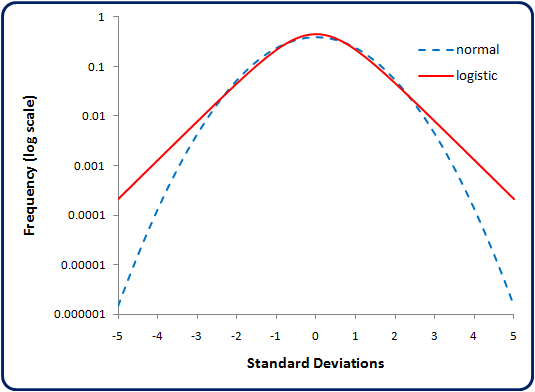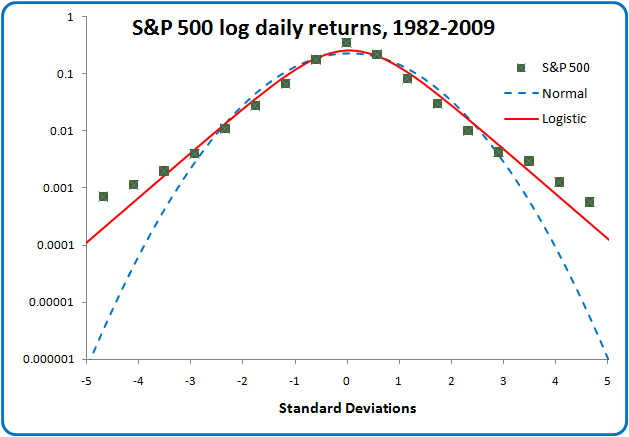### Fake data, part 1: The forgotten logistic distribution

May 2017: This is from my other blog that's no longer online. The original comments are no longer available, but you are welcome to add more.

Problem: We need to generate an endless stream of artificial market data, such that it maintains the statistical properties and behaviors of a real market.

Everybody is familiar with the normal (Gaussian) distribution. The classic bell curve that underlies many tools in statistics.

A normal distribution has a couple of glaring flaws, however:

• It requires numerical methods to calculate cumulative probability (area under the bell curve) and inverse of probability (i.e. for a known value of cumulative probability, what's the corresponding value of x?)
• It doesn't model many real-world situations well. Real-world distributions often have more outliers ("fatter tails" in the bell curve) than the normal distribution would suggest.

Fortunately, there's a simple alternative: The logistic distribution. Like the normal distribution, it is defined by two parameters, the mean μ and standard deviation σ. After playing around with it, I wonder why it isn't in wider use. It addresses the two concerns above:

• Cumulative and inverse cumulative probability functions are closed-form formulas, no numerical techniques required.
• The bell curve has somewhat fatter tails than the normal distribution.
Here's how they look overlayed. When plotted against a log scale, you can really see how much fatter the logistic distributions's tails are.So here are the formulas. The bell curve formula in both cases are pretty straightforward. The logistic distribution can be written in terms of either exponential or hyperbolic functions.

Probability distribution function (pdf)
Logistic $$f(x;\mu,s) = \frac{ e^{-(x-\mu)/s} }{s \left( 1+e^{-(x-\mu)/s} \right)^2} \\ \\ ~ \qquad =\frac{1}{4s} \mathrm{sech}^2 \left( \frac{x-\mu}{2s} \right ) \\ \\ ~ \qquad = \frac{\pi}{4\sigma\sqrt{3}} \mathrm{sech}^2 \left( \frac{\pi}{2\sqrt{3}} \frac{x-\mu}{\sigma} \right ) ~ \textrm{ using } ~ s=\frac{\sigma \sqrt{3}}{\pi}$$
Normal $$f(x;\mu,\sigma) = \frac{1}{\sigma\sqrt{2\pi}} e^{-(x-\mu)^2 /(2 \sigma^2)}$$

Actually, while the variance σ2 is defined for the logistic distribution, there isn't really something called a "standard deviation" as with the normal distribution. However, it is convenient to use that term for both, so that's what I'll do.

Okay, the pdf formulas are simple. But now when you want to calculate the cumulative probability, things get messy for the normal distribution, because you get into iterative numerical methods to solve the integral (although there's a good polynomial approximation that is widely used). The logistic cumulative distribution is a simple closed-form formula, which again may be expressed with a hyperbolic function.

Cumulative distribution function (cdf)
Logistic $$F(x;\mu,s) = \frac{1}{ 1+e^{-(x-\mu)/s}} \\ \\ ~ \qquad = \frac{1}{2} + \tanh \left ( \frac{x-\mu}{2s} \right )$$
Normal $$F(x;\mu,\sigma) = \frac{1}{\sigma\sqrt{2\pi}} \int_{-\infty}^{x} e^{-(t-\mu)^2 /(2 \sigma^2)}\,dt \\ \\ ~ \qquad \qquad =\frac{1}{2} \left[1+\mathrm{erf} \left(\frac{x-\mu} {\sigma\sqrt{2}} \right ) \right ]$$ (This must be solved numerically)

The situation is the same for the inverse case: The logistic distribution is simple to calculate, while the normal distribution is mathematically intractable.

Inverse cumulative distribution function
Logistic $$F^{-1}(p;\mu,s) = \mu + s \ln \left( \frac{p}{1+p} \right)$$
Normal (For a normal distribution, the inverse of the cumulative distribution must be solved numerically.)

So let's look at how it fits some real-world data.

Finaicial data is the easiest to get, and you can obtain a lot of it. So I downloaded the S&P 500 stock index history from 1982 through 2009, calculated the log returns, and plotted the distribution of log returns as a function of the number of standard deviations from the mean. The squares represent the bin value for the actual S&P data. The red line is the logistic distribution and the dashed blue line is the normal distribution. Both distributions use the mean and standard deviation (µ and σ) calculated from the S&P 500 returns.Neither distribution captures the sharpness of the peak in the actual data, but the logistic distribution has a higher narrower peak than the normal distribution, so it's a closer approximation to this real-world data.

Now let's look at how the tails of each distribution fit the data.Look at that! The S&P 500 returns have significantly fatter tails than the normal distribution is capable of modeling. There's an order of magnitude difference at about 3.5 standard deviations from the mean, and the normal distribution is nearly 3 orders of magnitude off the mark at 5 standard deviations out.

In contrast, the logistic distribution fits the data fairly well over 10 sigma. However, you can see it diverging there at the ends. Sure enough, when you increase the horizontal scale to 20 standard deviations wide, you see that both distributions break down.Okay, the logistic distribution isn't a great fit way out at the extremes of the tails. At least it fits the curve for 5 standard deviations around the mean. The normal distribution fares much worse, though. I found I could force-fit the logistic distribution to the data by substituting (x−μ)/s with π sinh−1[(x−μ)/(πs)], but that pdf doesn't appear to be integrable either, except by numerical methods.

It is interesting to note that stock market returns have what appear to be 9-sigma events, far, far more frequently (over a million times more frequently) than the Gaussian bell curve would suggest. These are the "black swan" events that wipe out investment managers who use standard statistical tools having an underlying assumption that everything is normally distributed.

It would seem that there should be a way to profit from events like this that occur more frequently than expected.